NCERT Solutions Chapter 8 - Quadrilaterals (I), Class 9, Maths

# NCERT Solutions Chapter 8 - Quadrilaterals (I), Class 9, Maths - Extra Documents & Tests for Class 9

Exercise 8.1

1. The angles of quadrilateral are in the ratio 3 : 5 : 9 : 13. Find all the angles of the quadrilateral.

Let x be the common ratio between the angles.
Sum of the interior angles of the quadrilateral = 360°
Now,
3x + 5x + 9x + 13x = 360°
⇒ 30x = 360°
⇒ x = 12°
3x = 3×12° = 36°
5x = 5×12° = 60°
9x = 9×12° = 108°
13x = 13×12° = 156°

2. If the diagonals of a parallelogram are equal, then show that it is a rectangle.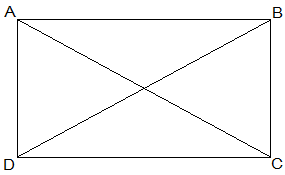Given,
AC = BD
To show,
To show ABCD is a rectangle we have to prove that one of its interior angle is right angled.
Proof,
BC = BA (Common)
AC = AD (Opposite sides of a parallelogram are equal)
AC = BD (Given)
Therefore, ΔABC ≅ ΔBAD by SSS congruence condition.
∠A = ∠B (by CPCT)
also,
∠A + ∠B = 180° (Sum of the angles on the same side of the transversal)
⇒ 2∠A = 180°
⇒ ∠A = 90°
Thus ABCD is a rectangle.

3. Show that if the diagonals of a quadrilateral bisect each other at right angles, then it is a rhombus.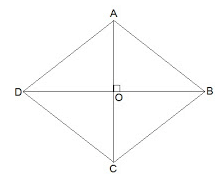Let ABCD be a quadrilateral whose diagonals bisect each other at right angles.
Given,
OA = OC, OB = OD and ∠AOB = ∠BOC = ∠OCD = ∠ODA = 90°
To show,
ABCD is parallelogram and AB = BC = CD = AD
Proof,
In ΔAOB and ΔCOB,
OA = OC (Given)
∠AOB = ∠COB (Opposite sides of a parallelogram are equal)
OB = OB (Common)
Therefore, ΔAOB ≅ ΔCOB by SAS congruence condition.
Thus, AB = BC (by CPCT)
Similarly we can prove,
AB = BC = CD = AD
Opposites sides of a quadrilateral are equal hence ABCD is a parallelogram.
Thus, ABCD is rhombus as it is a parallelogram whose diagonals intersect at right angle.

4. Show that the diagonals of a square are equal and bisect each other at right angles.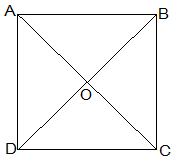Let ABCD be a square and its diagonals AC and BD intersect each other at O.
To show,
AC = BD, AO = OC and ∠AOB = 90°
Proof,
BC = BA (Common)
Therefore, ΔABC ≅ ΔBAD by SAS congruence condition.
Thus, AC = BD by CPCT. Therefore, diagonals are equal.
Now,
In ΔAOB and ΔCOD,
∠BAO = ∠DCO (Alternate interior angles)
∠AOB = ∠COD (Vertically opposite)
AB = CD (Given)
Therefore, ΔAOB ≅ ΔCOD by AAS congruence condition.
Thus, AO = CO by CPCT. (Diagonal bisect each other.)
Now,
In ΔAOB and ΔCOB,
OB = OB (Given)
AO = CO (diagonals are bisected)
AB = CB (Sides of the square)
Therefore, ΔAOB ≅ ΔCOB by SSS congruence condition.
also, ∠AOB = ∠COB
∠AOB + ∠COB = 180° (Linear pair)
Thus, ∠AOB = ∠COB = 90° (Diagonals bisect each other at right angles)

5. Show that if the diagonals of a quadrilateral are equal and bisect each other at right angles, then it is a square.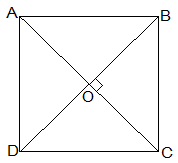Given,
Let ABCD be a quadrilateral in which diagonals AC and BD bisect each other at right angle at O.
To prove,
Proof,
In ΔAOB and ΔCOD,
AO = CO (Diagonals bisect each other)
∠AOB = ∠COD (Vertically opposite)
OB = OD (Diagonals bisect each other)
Therefore, ΔAOB ≅ ΔCOD by SAS congruence condition.
Thus, AB = CD by CPCT. --- (i)
also,
∠OAB = ∠OCD (Alternate interior angles)
⇒ AB || CD
Now,
In ΔAOD and ΔCOD,
AO = CO (Diagonals bisect each other)
∠AOD = ∠COD (Vertically opposite)
OD = OD (Common)
Therefore, ΔAOD ≅ ΔCOD by SAS congruence condition.
Thus, AD = CD by CPCT. --- (ii)
also,
⇒ AD = BC = CD = AB --- (ii)
also,  ∠ADC = ∠BCD  by CPCT.
and ∠ADC + ∠BCD = 180° (co-interior angles)
⇒ ∠ADC = 90° --- (iii)
One of the interior ang is right angle.
Thus, from (i), (ii) and (iii) given quadrilateral ABCD is a square.

6. Diagonal AC of a parallelogram ABCD bisects ∠A (see Fig. 8.19). Show that
(i) it bisects ∠C also,
(ii) ABCD is a rhombus.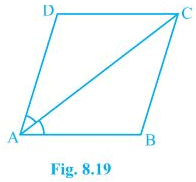(i)
AD = CB (Opposite sides of a ||gm)
DC = BA (Opposite sides of a ||gm)
AC = CA (Common)
Therefore, ΔADC ≅ ΔCBA by SSS congruence condition.
Thus,
∠ACD = ∠CAB by CPCT
⇒ ∠ACD = ∠BCA
Thus, AC bisects ∠C also.

⇒ AD = CD (Opposite sides of equal angles of a triangle are equal)
Also, AB = BC = CD = DA (Opposite sides of a ||gm)
Thus, ABCD is a rhombus.

7. ABCD is a rhombus. Show that diagonal AC bisects ∠A as well as ∠C and diagonal BD bisects ∠B as well as ∠D.Let ABCD is a rhombus and AC and BD are its diagonals.
Proof,
AD = CD (Sides of a rhombus)
∠DAC = ∠DCA (Angles opposite of equal sides of a triangle are equal.)
also, AB || CD
⇒ ∠DAC = ∠BCA (Alternate interior angles)
⇒ ∠DCA = ∠BCA
Therefore, AC bisects ∠C.
Similarly, we can prove that diagonal AC bisects ∠A.

Also, by preceding above method we can prove that diagonal BD bisects ∠B as well as ∠D.

8. ABCD is a rectangle in which diagonal AC bisects ∠A as well as ∠C. Show that:
(i) ABCD is a square
(ii) diagonal BD bisects ∠B as well as ∠D.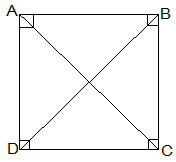(i)∠DAC = ∠DCA (AC bisects ∠A as well as ∠C)
⇒ AD = CD (Sides opposite to equal angles of a triangle are equal)
also, CD = AB (Opposite sides of a rectangle)
Therefore, AB = BC = CD = AD
Thus, ABCD is a square.

(ii) In ΔBCD,
BC = CD
⇒ ∠CDB = ∠CBD (Angles opposite to equal sides are equal)
also, ∠CDB = ∠ABD (Alternate interior angles)
⇒ ∠CBD = ∠ABD
Thus, BD bisects ∠B
Now,
Thus, BD bisects ∠D

9. In parallelogram ABCD, two points P and Q are taken on diagonal BD such that DP = BQ (see Fig. 8.20). Show that:
(i) ΔAPD ≅ ΔCQB
(ii) AP = CQ
(iii) ΔAQB ≅ ΔCPD
(iv) AQ = CP
(v) APCQ is a parallelogram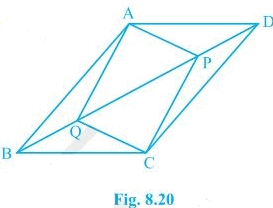(i) In ΔAPD and ΔCQB,
DP = BQ (Given)
∠ADP = ∠CBQ (Alternate interior angles)
AD = BC (Opposite sides of a ||gm)
Thus, ΔAPD ≅ ΔCQB by SAS congruence condition.

(ii) AP = CQ by CPCT as ΔAPD ≅ ΔCQB.

(iii) In ΔAQB and ΔCPD,
BQ = DP (Given)
∠ABQ = ∠CDP (Alternate interior angles)
AB = BCCD (Opposite sides of a ||gm)
Thus, ΔAQB ≅ ΔCPD by SAS congruence condition.

(iv) AQ = CP by CPCT as ΔAQB ≅ ΔCPD.

(v) From (ii)  and (iv), it is clear that APCQ has equal opposite sides also it has equal opposite angles. Thus, APCQ is a ||gm.

10. ABCD is a parallelogram and AP and CQ are perpendiculars from vertices A and C on diagonal BD (see Fig. 8.21). Show that
(i) ΔAPB ≅ ΔCQD
(ii) AP = CQ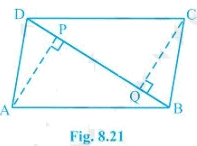(i) In ΔAPB and ΔCQD,
∠ABP = ∠CDQ (Alternate interior angles)
∠APB = ∠CQD (equal to right angles as AP and CQ are perpendiculars)
AB = CD (ABCD is a parallelogram)
Thus, ΔAPB ≅ ΔCQD by AAS congruence condition.

(ii) AP = CQ by CPCT as ΔAPB ≅ ΔCQD.

11. In ΔABC and ΔDEF, AB = DE, AB || DE, BC = EF and BC || EF. Vertices A, B and C are joined to vertices D, E and F respectively (see Fig. 8.22).
Show that
(i) quadrilateral ABED is a parallelogram
(ii) quadrilateral BEFC is a parallelogram
(iv) quadrilateral ACFD is a parallelogram
(v) AC = DF
(vi) ΔABC ≅ ΔDEF.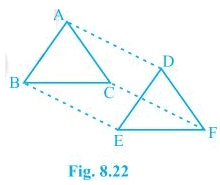(i) AB = DE and AB || DE (Given)

Thus, quadrilateral ABED is a parallelogram because two opposite sides of a quadrilateral are equal and parallel to each other.

(ii) Again BC = EF and BC || EF.

Thus, quadrilateral BEFC is a parallelogram.

(iii)  Since ABED and BEFC are parallelograms.
⇒ AD = BE and BE = CF (Opposite sides of a parallelogram are equal)

Also, AD || BE and BE || CF (Opposite sides of a parallelogram are parallel)

(iv) AD and CF are opposite sides of quadrilateral ACFD which are equal and parallel to each other. Thus, it is a parallelogram.

(v) AC || DF and AC = DF because ACFD is a parallelogram.

(vi) In ΔABC and ΔDEF,

AB = DE (Given)

BC = EF (Given)

AC = DF (Opposite sides of a parallelogram)

Thus, ΔABC ≅ ΔDEF by SSS congruence condition.

12. ABCD is a trapezium in which AB || CD and

AD = BC (see Fig. 8.23). Show that
(i) ∠A = ∠B
(ii) ∠C = ∠D
(iv) diagonal AC = diagonal BD

[Hint : Extend AB and draw a line through C parallel to DA intersecting AB produced at E.]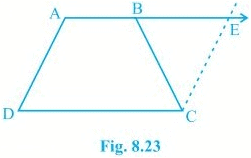Construction: Draw a line through C parallel to DA intersecting AB produced at E.
(i) CE = AD (Opposite sides of a parallelogram)
Therefor, BC = CE
⇒ ∠CBE = ∠CEB
also,
∠A + ∠CBE = 180° (Angles on the same side of transversal and ∠CBE = ∠CEB)
∠B + ∠CBE = 180° (Linear pair)
⇒ ∠A = ∠B

(ii) ∠A + ∠D = ∠B + ∠C = 180° (Angles on the same side of transversal)
⇒ ∠A + ∠D = ∠A + ∠C (∠A = ∠B)
⇒ ∠D = ∠C

AB = AB (Common)
∠DBA = ∠CBA
Thus, ΔABC ≅ ΔBAD by SAS congruence condition.

(iv) Diagonal AC = diagonal BD by CPCT as ΔABC ≅ ΔBA.

The document NCERT Solutions Chapter 8 - Quadrilaterals (I), Class 9, Maths | Extra Documents & Tests for Class 9 is a part of the Class 9 Course Extra Documents & Tests for Class 9.
All you need of Class 9 at this link: Class 9

## Extra Documents & Tests for Class 9

1 videos|228 docs|21 tests

## FAQs on NCERT Solutions Chapter 8 - Quadrilaterals (I), Class 9, Maths - Extra Documents & Tests for Class 9

 1. What are the properties of a quadrilateral?Ans. A quadrilateral is a polygon with four sides. The properties of a quadrilateral include the sum of its interior angles being equal to 360 degrees, opposite angles being equal, opposite sides being parallel, and consecutive angles being supplementary.
 2. How do you classify quadrilaterals based on their sides and angles?Ans. Quadrilaterals can be classified based on their sides as follows: - A quadrilateral with all sides of equal length is called a square. - A quadrilateral with opposite sides parallel and equal in length is called a parallelogram. - A quadrilateral with exactly one pair of parallel sides is called a trapezium. Quadrilaterals can also be classified based on their angles as follows: - A quadrilateral with all interior angles measuring 90 degrees is called a rectangle. - A quadrilateral with exactly one pair of opposite angles measuring 90 degrees is called a kite. - A quadrilateral with all interior angles measuring less than 90 degrees is called a rhombus.
 3. How do you find the area of a quadrilateral?Ans. The area of a quadrilateral can be determined using different formulas based on the type of quadrilateral. Here are the formulas for some common quadrilaterals: - Square: Area = side length * side length - Rectangle: Area = length * width - Parallelogram: Area = base length * height - Trapezium: Area = (sum of parallel sides) * height / 2 - Rhombus: Area = (product of diagonals) / 2
 4. Can a quadrilateral have all angles measuring 90 degrees?Ans. Yes, a quadrilateral can have all angles measuring 90 degrees. This type of quadrilateral is called a rectangle. In a rectangle, all four interior angles are right angles, and opposite sides are equal in length.
 5. How do you prove that a quadrilateral is a parallelogram?Ans. To prove that a quadrilateral is a parallelogram, you can use any of the following methods: - Show that both pairs of opposite sides are parallel. - Show that both pairs of opposite sides are equal in length. - Show that one pair of opposite sides is both parallel and equal in length. - Show that both pairs of opposite angles are equal. - Show that one pair of opposite angles is both equal and supplementary. By demonstrating any of these properties, you can establish that a quadrilateral is a parallelogram.

## Extra Documents & Tests for Class 9

1 videos|228 docs|21 testsExplore Courses for Class 9 examSignup to see your scores go up within 7 days! Learn & Practice with 1000+ FREE Notes, Videos & Tests.
10M+ students study on EduRev
Track your progress, build streaks, highlight & save important lessons and more!
Related Searches

,

,

,

,

,

,

,

,

,

,

,

,

,

,

,

,

,

,

,

,

,

,

,

,

,

,

,

;